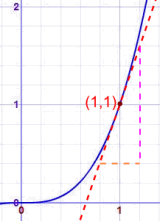# 微积分入门小山和小李开车去出旅行……但车速表坏了。
 小李： "小山！我们现在开得多快？" 小山: "等一等……" "上一分钟我们走了 1.2 千米，所以车速是：" 1.2 千米m/分钟 × 一小时 60 分钟 = 每小时 72 千米 小李: "不，小山! 我不是问上一分钟或上一秒的平均速度，我想知道我们现在的速度。" 小山: "那么我们在这里测量……这个路标……来！""好了，我们在路标待了零秒，走了……零米！" 速度是 0米 / 0秒 = 0/0 = 我不知道是多少！ "小山，我没招了！我要知道在某个时间里走的某个距离，才能计算速度。但你说时间是零？不可能！"

## 那……可不可以求一个相当准确的近似值？### "起跳一秒后你会下落得多快？"

d = 5t2

• d = 跌了的距离（米）
• t = 起跳后的时间（秒）

（注意：这个公式是从物体在 引力影响下，下落距离的公式：d = ½gt2 简化而成的。）

d = 5t2 = 5 × 12 = 5 m

 速度 = 距离 时间

1秒后：

 速度 = 5米 = 5米每秒 1秒

"可是，"小李说，"这也是从你起跳开始的平均速度，…… 我想知道的是刚好在 1秒时的速度，好让我准备好我的相机。"速度 = 5 − 5米 = 0米 = ???? 1 − 1秒 0秒s

1秒 ，小山落下了

d = 5t2 = 5 × (1)2 = 5 m

(1+Δt) 秒，小山落下了

d = 5t2 = 5 × (1+Δt)2 m

 (1+Δt)2 = (1+Δt)(1+Δt) = 1 + 2Δt + (Δt)2

 d = 5 × (1+2Δt+(Δt)2) m = 5 + 10Δt + 5(Δt)2 md 的改变 = (5 + 10Δt + 5(Δt)2) − 5米 = 10Δt + 5(Δt)2米

 速率 = 10Δt +5(Δt)2米 Δt s = 10 + 5Δt 米每秒### 例子：函数 y = x3 在 x=1 的坡度是多少？

y = 1 + 3Δx + 3(Δx)2 + (Δx)3

y 从 x = 1 到 x = 1+Δx 的差值是：

 y 的改变 = 1 + 3Δx + 3(Δx)2 + (Δx)3 − 1 = 3Δx + 3(Δx)2 + (Δx)3

 坡度 = 3Δx + 3(Δx)2 + (Δx)3 Δx = 3 + 3Δx + (Δx)2

 这是 y = x3 的图   坡度持续地改变，但在 (1,1) 这点，我们可以画一条切线， 然后算出在那一点的坡度是等于 3的。 （你可以数数格子来看看！）